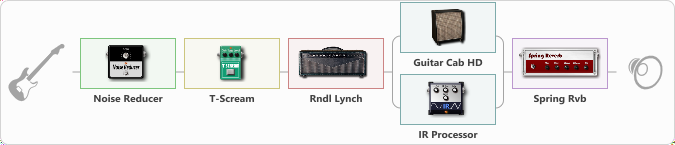# Carlinos33

Discussion in 'ToneLib-GFX presets' started by carlinos3, Aug 26, 2023.

1. Carlinos33

Preset name: Boozed and Broozed

Effects chain:Effect: "Noise Reducer" (Dynamics / Filter), active - "yes"
{
"Sens" = 57
"Mode" = Hard
}

Effect: "T-Scream" (Overdrive / Distortion), active - "yes"
{
"Drive" = 35
"Tone" = 80
"Level" = 90
}

Effect: "Rndl Lynch" (Amp simulators), active - "yes"
{
"Gain" = 100
"Bass" = 52
"Middle" = 35
"Treble" = 65
"Presence" = 90
"Master" = 100
"Level (dB)" = 0
}

Effect: "Splitter" (Dynamics / Filter)
{
"A-Bypass" = Off
"A-Pan" = 0
"A-Level" = 45
"B-Bypass" = Off
"B-Pan" = 3
"B-Level" = 62
"Width" = 0

'A' branch:
{

Effect: "Guitar Cab HD" (Cabinets), active - "yes"
{
"Model" = Matchless DC30 (2x12")
"Mic Model" = Ribbon M160
"Mic Position" = Center
"Mic Distance" = Near
"Low Cut (Hz)" = 80
"Hi Cut (kHz)" = 20.0
"Mix" = 100
"Level (dB)" = -6
}
}
'B' branch:
{

Effect: "IR Processor" (Cabinets), active - "yes"
{
"IR" = seoane_dc
"Low Cut (Hz)" = 0
"Hi Cut (kHz)" = 20.0
"Mix" = 100
"Level (dB)" = 0
}
}
}

Effect: "Spring Rvb" (Reverberation), active - "yes"
{
"Time" = 7.0
"PreDelay" = 0
"LoDamp" = 16
"HiDamp" = 26
"Mix" = 43
}

Note: You will need to download and install the ToneLib-GFX software to use the preset.

#### Attached Files:

• ###### Carlinos33.tlgfx
File size:
81.1 KB
Views:
670
Daniel_K145 likes this.